# RS Aggarwal Solutions for Class 8 Maths Chapter 9 - Percentage

RS Aggarwal Solutions for Class 8 Maths Chapter 9- Percentage, are provided here. Our expert faculty team has prepared solutions in order to help you with your exam preparation to acquire good marks in Maths. RS Aggarwal Solutions book for Class 8 Maths comes in very handy at this point. Our solution module utilizes various shortcut tips and practical examples to explain all the exercise questions in a simple and easily understandable language. If you wish to secure an excellent score, solving RS Aggarwal Solutions for Class 8 is an utmost necessity. These solutions will help you in gaining knowledge and strong command over the subject. Practising the textbook questions will help you in analyzing your level of preparation and knowledge of the concept.

In order to help you understand and solve the problems, we at BYJU’S have designed these solutions in a very lucid and clear manner that helps students solve problems in the most efficient possible ways. Here is the RS Aggarwal Solutions for Class 8 Chapter 9 where solutions are solved in detail. Download pdf of Class 8 Chapter 9 in their respective links.

## Download PDF of RS Aggarwal Solutions for Class 8 Maths Chapter 9 – Percentage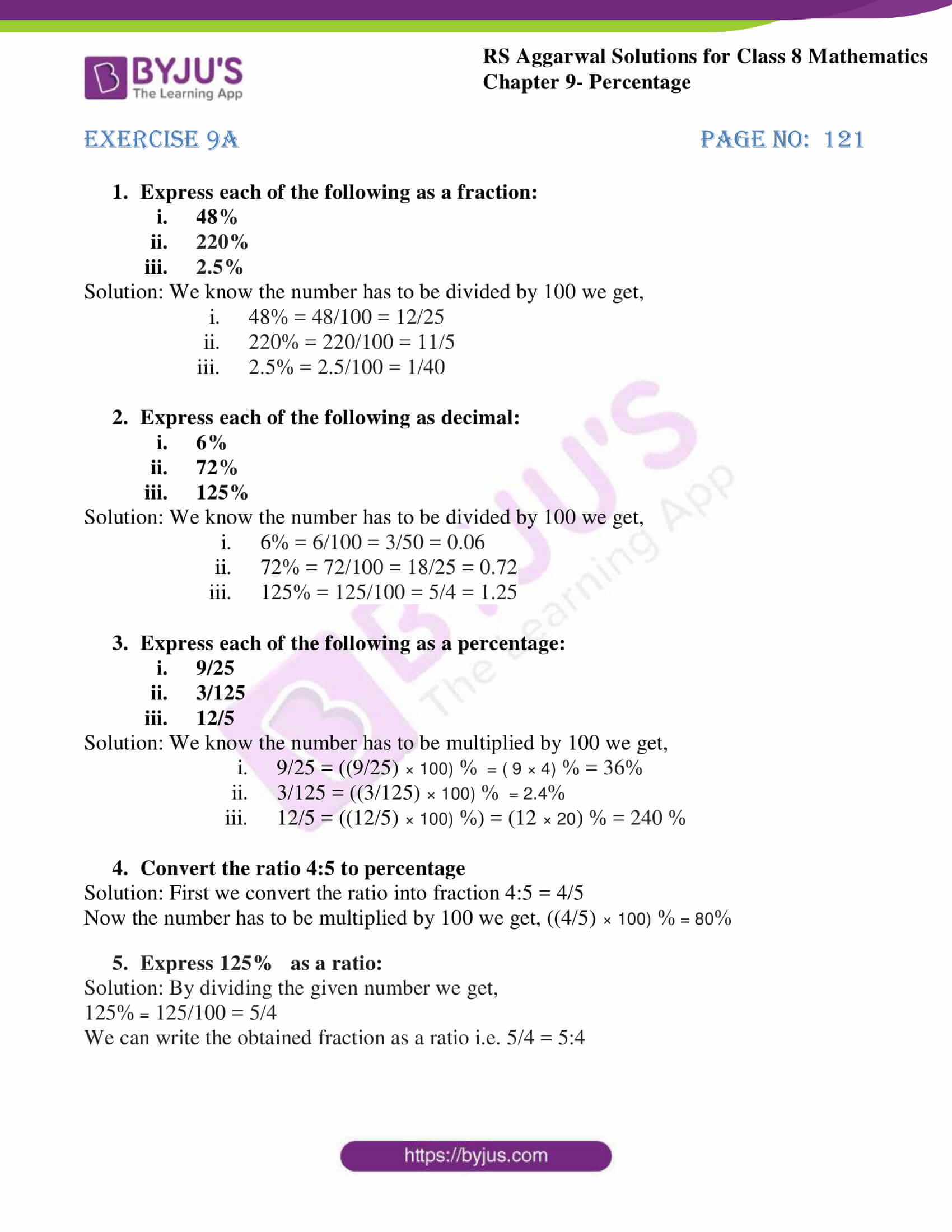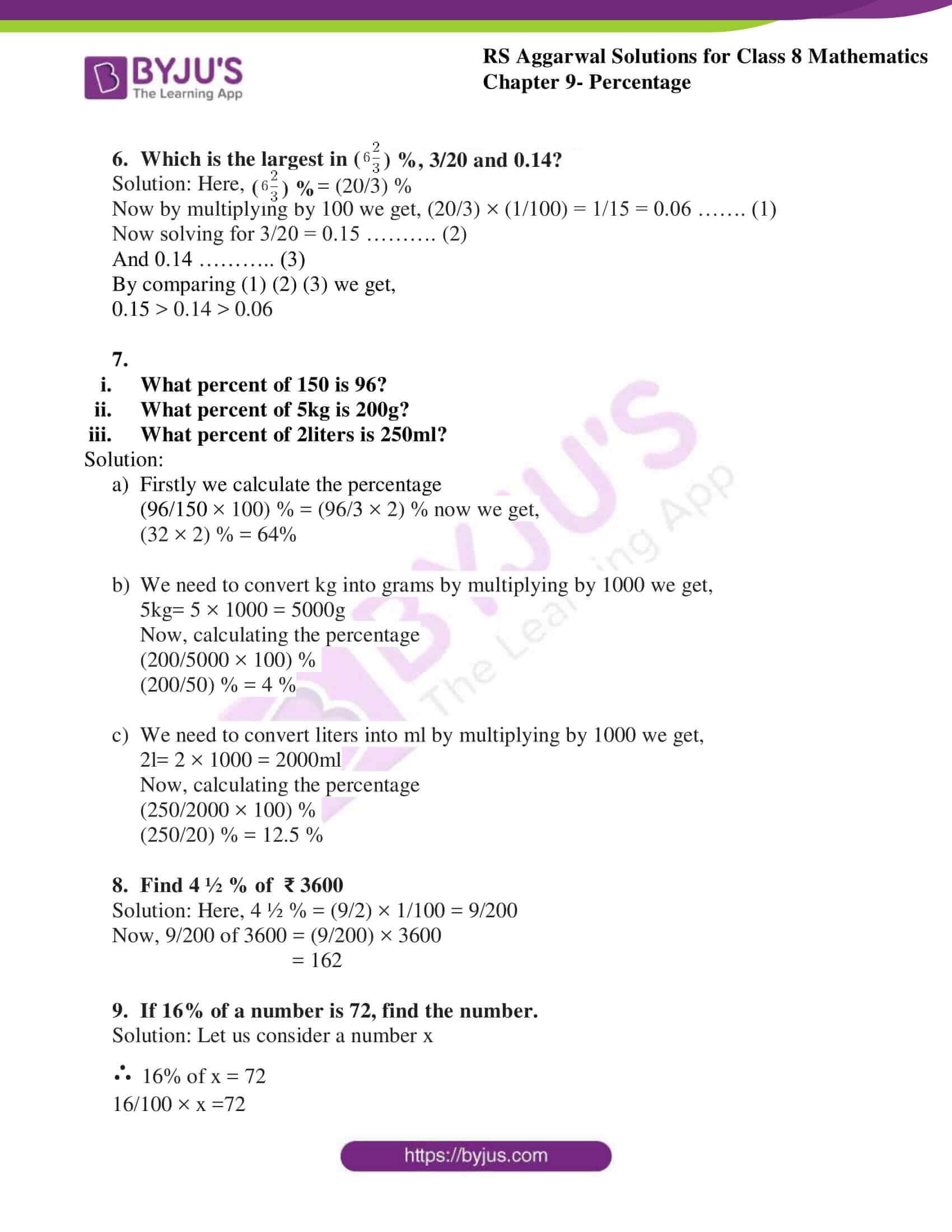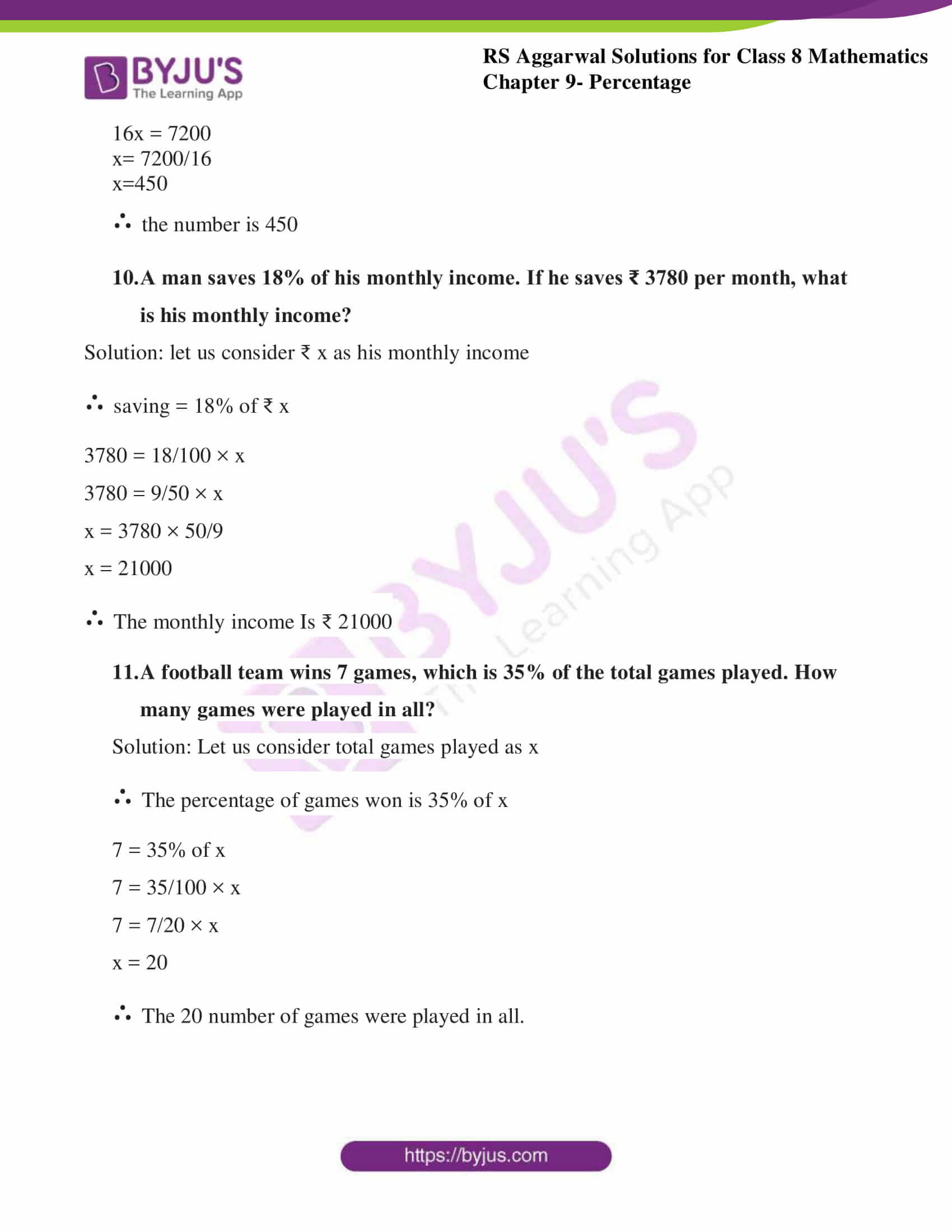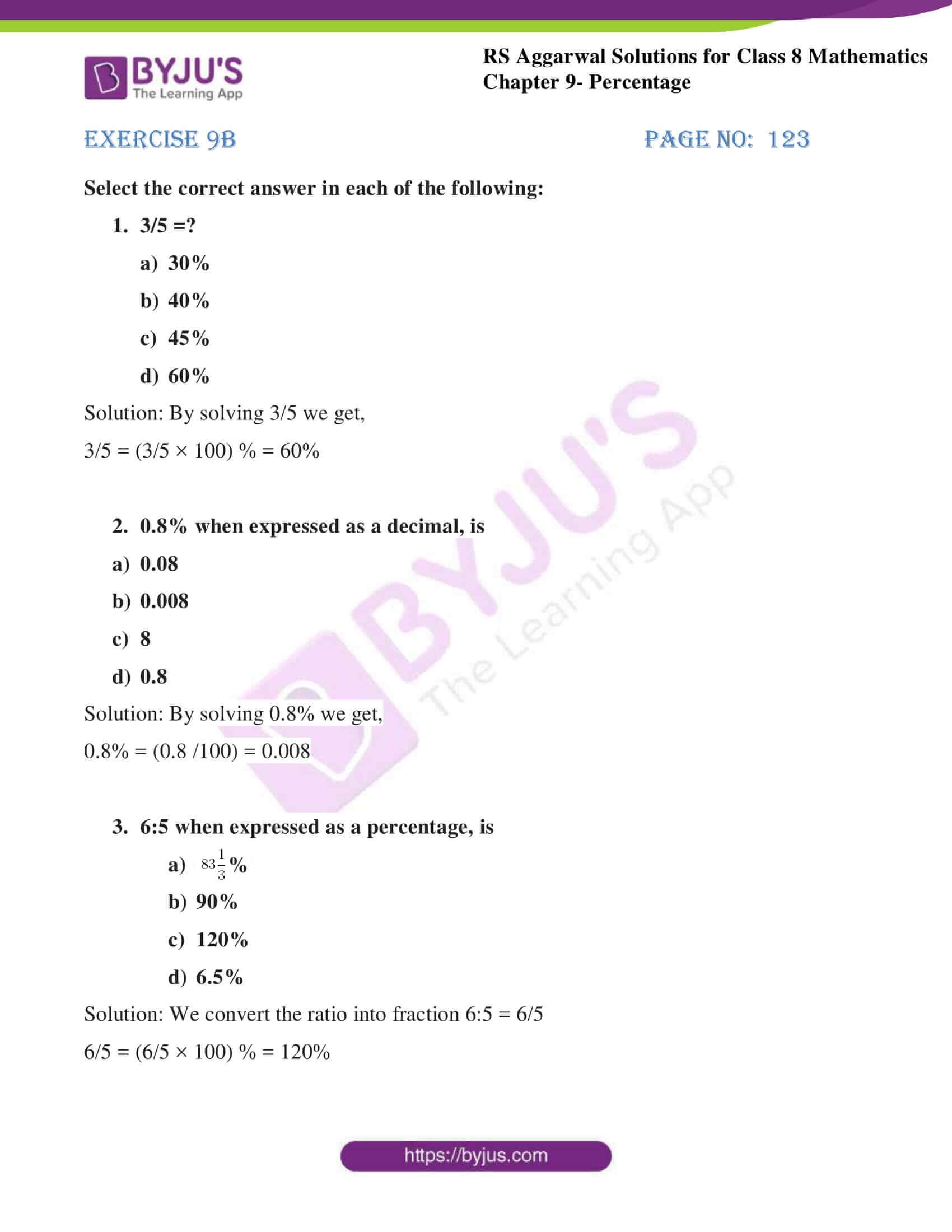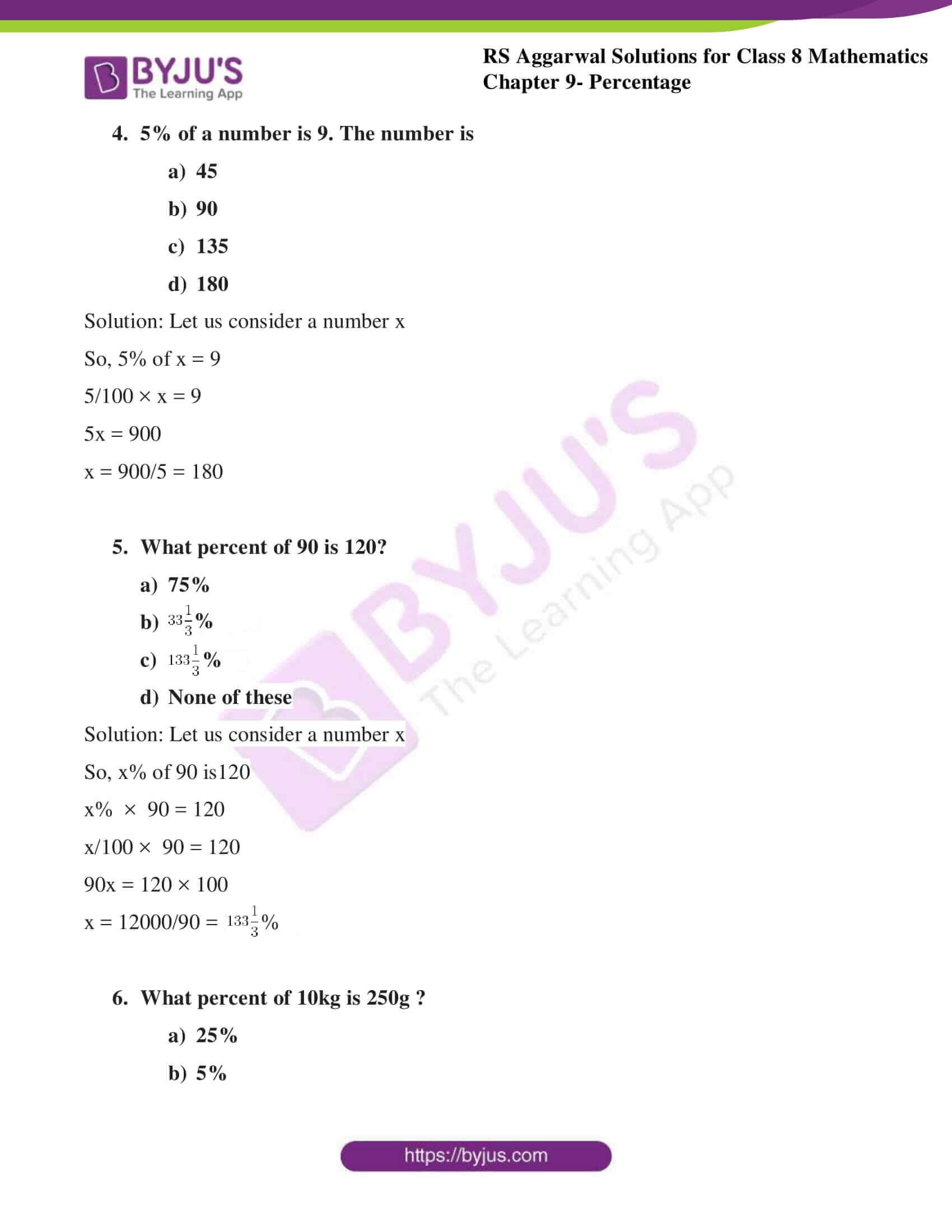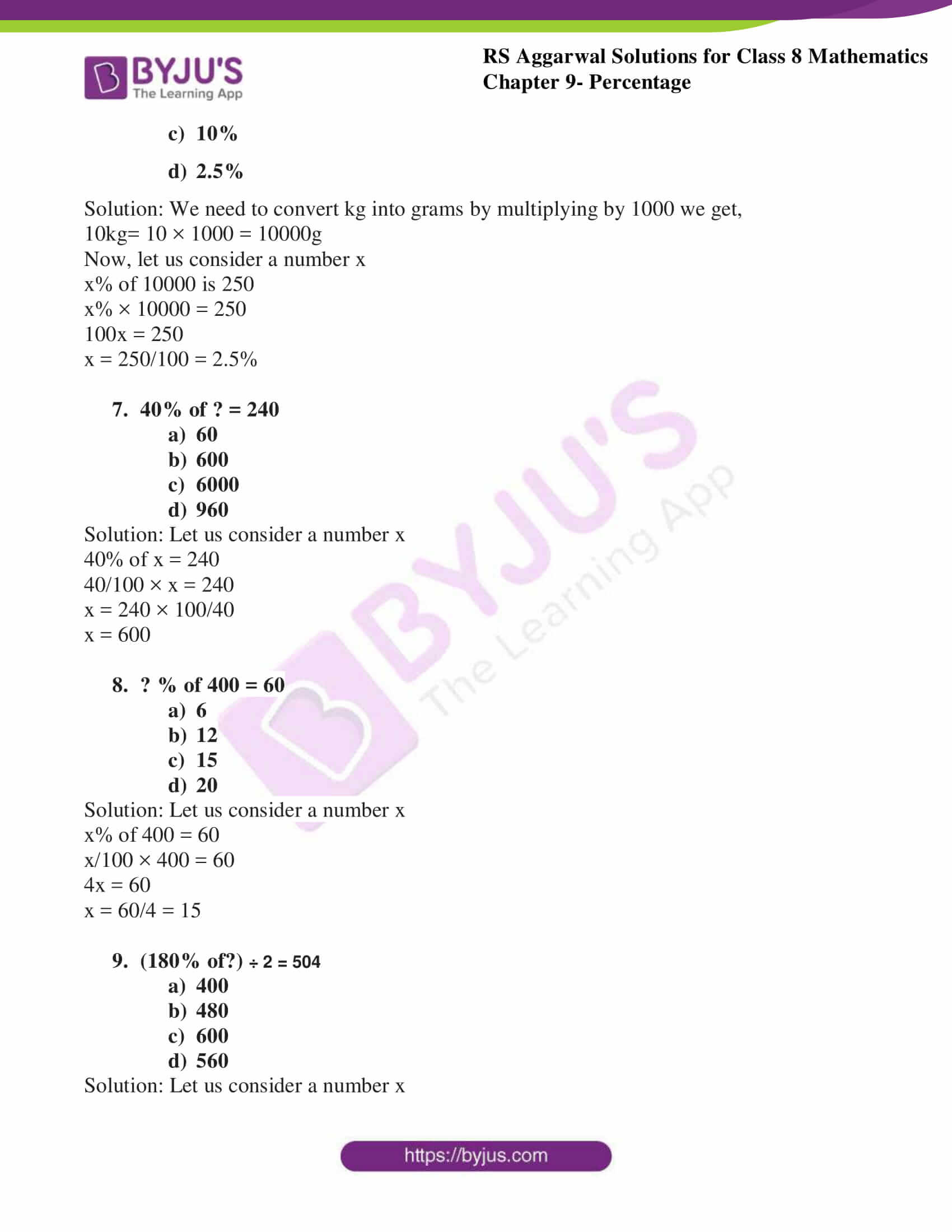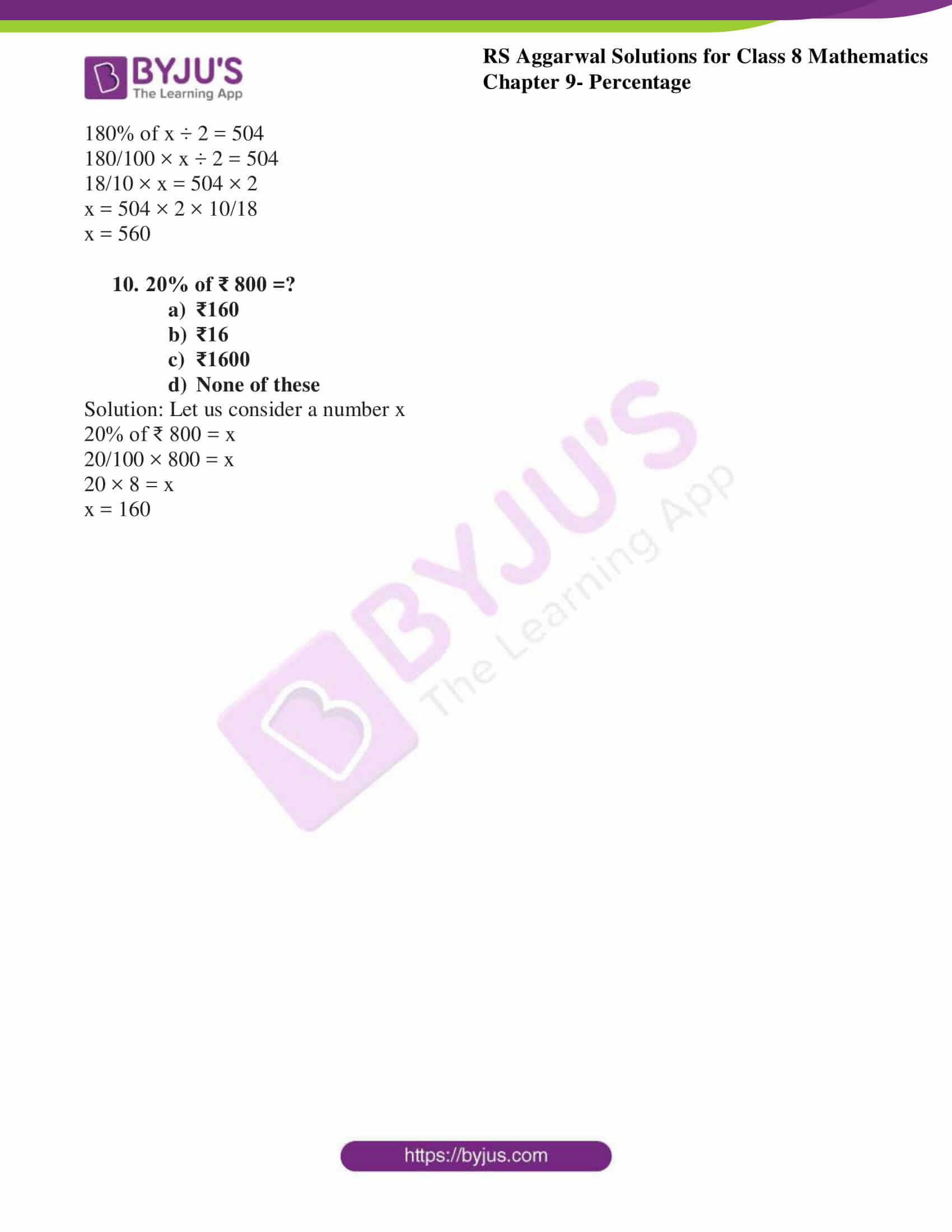## Exercise 9A

1. Express each of the following as a fraction:

1. 48%
2. 220%
3. 2.5%

Solution: We know the number has to be divided by 100 we get,

1. 48% = 48/100 = 12/25
2. 220% = 220/100 = 11/5
3. 2.5% = 2.5/100 = 1/40

2. Express each of the following as decimal:

1. 6%
2. 72%
3. 125%

Solution: We know the number has to be divided by 100 we get,

1. 6% = 6/100 = 3/50 = 0.06
2. 72% = 72/100 = 18/25 = 0.72
3. 125% = 125/100 = 5/4 = 1.25

3. Express each of the following as a percentage:

1. 9/25
2. 3/125
3. 12/5

Solution: We know the number has to be multiplied by 100 we get,

1. 9/25 = ((9/25) × 100) % = ( 9 × 4) % = 36%
2. 3/125 = ((3/125) × 100) % = 2.4%
3. 12/5 = ((12/5) × 100) %) = (12 × 20) % = 240 %

4. Convert the ratio 4:5 to percentage

Solution: First we convert the ratio into fraction 4:5 = 4/5

Now the number has to be multiplied by 100 we get, ((4/5) × 100) % = 80%

5. Express 125% as a ratio:

Solution: By dividing the given number we get,

125% = 125/100 = 5/4

We can write the obtained fraction as a ratio i.e. 5/4 = 5:4

6. Which is the largest in ($6\frac{2}{3}$) %, 3/20 and 0.14?

Solution: Here, ($6\frac{2}{3}$) % = (20/3) %

Now by multiplying by 100 we get, (20/3) × (1/100) = 1/15 = 0.06 ……. (1)

Now solving for 3/20 = 0.15 ………. (2)

And 0.14 ……….. (3)

By comparing (1) (2) (3) we get,

0.15 > 0.14 > 0.06

7. find:

1. What percent of 150 is 96?
2. What percent of 5kg is 200g?
3. What percent of 2liters is 250ml?

Solution:

1. Firstly we calculate the percentage(96/150 × 100) % = (96/3 × 2) % now we get,(32 × 2) % = 64%
2. We need to convert kg into grams by multiplying by 1000 we get,5kg= 5 × 1000 = 5000gNow, calculating the percentage(200/5000 × 100) %(200/50) % = 4 %
3. We need to convert liters into ml by multiplying by 1000 we get,2l= 2 × 1000 = 2000mlNow, calculating the percentage(250/2000 × 100) %(250/20) % = 12.5 %

8. Find 4 ½ % of ₹ 3600

Solution: Here, 4 ½ % = (9/2) × 1/100 = 9/200

Now, 9/200 of 3600 = (9/200) × 3600

= 162

9. If 16% of a number is 72, find the number.

Solution: Let us consider a number x

∴ 16% of x = 72

16/100 × x =72

16x = 7200

x= 7200/16

x=450

∴ the number is 450

10. A man saves 18% of his monthly income. If he saves ₹ 3780 per month, what is his monthly income?

Solution: let us consider ₹ x as his monthly income

∴ saving = 18% of ₹ x

3780 = 18/100 × x

3780 = 9/50 × x

x = 3780 × 50/9

x = 21000

∴ The monthly income Is ₹ 21000

11. A football team wins 7 games, which is 35% of the total games played. How many games were played in all?

Solution: Let us consider total games played as x

∴ The percentage of games won is 35% of x

7 = 35% of x

7 = 35/100 × x

7 = 7/20 × x

x = 20

∴ The 20 number of games were played in all.

## Exercise 9B

Select the correct answer in each of the following:

1. 3/5 =?

1. 30%
2. 40%
3. 45%
4. 60%

Solution:

By solving 3/5 we get,3/5 = (3/5 × 100) % = 60%

2. 0.8% when expressed as a decimal, is

1. 0.08
2. 0.008
3. 8
4. 0.8

Solution:

By solving 0.8% we get,0.8% = (0.8 /100) = 0.008

3. 6:5 when expressed as a percentage, is

1. $83\frac{1}{3}$%
2. 90%
3. 120%
4. 6.5%

Solution:

We convert the ratio into fraction 6:5 = 6/56/5 = (6/5 × 100) % = 120%

4. 5% of a number is 9. The number is

1. 45
2. 90
3. 135
4. 180

Solution:

Let us consider a number xSo, 5% of x = 95/100 × x = 95x = 900x = 900/5 = 180

5. What percent of 90 is 120?

1. 75%
2. $33\frac{1}{3}$%
3. $133\frac{1}{3}$%
4. None of these

Solution:

Let us consider a number x

So, x% of 90 is 120

x% × 90 = 120x/100 × 90 = 120

90x = 120 × 100x = 12000/90 = $133\frac{1}{3}$%

6. What percent of 10kg is 250g ?

1. 25%
2. 5%
3. 10%
4. 2.5%

Solution:

We need to convert kg into grams by multiplying by 1000

we get,10kg= 10 × 1000 = 10000g

Now, let us consider a number xx% of 10000 is 250x% × 10000 = 250100x = 250x = 250/100 = 2.5%

7. 40% of ? = 240

1. 60
2. 600
3. 6000
4. 960

Solution:

Let us consider a number x

40% of x = 24040/100 × x = 240x = 240 × 100/40x = 600

8. ? % of 400 = 60

1. 6
2. 12
3. 15
4. 20

Solution:

Let us consider a number x

x% of 400 = 60x/100 × 400 = 604x = 60x = 60/4 = 15

9. (180% of?) ÷ 2 = 504

1. 400
2. 480
3. 600
4. 560

Solution:

Let us consider a number x

180% of x ÷ 2 = 504

180/100 × x ÷ 2 = 504

18/10 × x = 504 × 2x = 504 × 2 × 10/18x = 560

10. 20% of ₹ 800 =?

1. ₹160
2. ₹16
3. ₹1600
4. None of these

Solution: Let us consider a number x

20% of ₹ 800 = x

20/100 × 800 = x

20 × 8 = x

x = 160

## RS Aggarwal Solutions for Class 8 Maths Chapter 9 – Percentage

Chapter 9- Percentage contains 2 exercises and the RS Aggarwal solutions given on this page provide the solutions for the questions present in each exercise. Now, let us have a look at the concepts discussed in this chapter.

• Expressing percentage into fraction and vice-versa.
• Convert ratio into percentage and vice-versa.

Exercise 9A

Exercise 9B

### Chapter Brief of RS Aggarwal Solutions for Class 8 Maths Chapter 9 – Percentage

RS Aggarwal Solutions for Class 8 Maths Chapter 9 – Percentage, ensures that the students are thorough and familiar with the concepts. Regular revision of important concepts and formulas over time to time is the best way to strengthen your concepts. The solutions are designed in such a way that they are easy to understand and solve.

As the Chapter is about Percentage, we use Percentage to compare values, quantify changes, to calculate the amount represented by an increase or decrease and most widely used to calculate the financial aspects of everyday life. In the RS Aggarwal Solutions book, many such exercise problems are given, which enhance familiarity with the concepts.